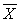# [Solved] TABLE 10-15 The Table Below Presents the Summary Statistics for the Starting

Question 206
Multiple Choice

## TABLE 10-15The table below presents the summary statistics for the starting annual salaries (in thousands of dollars) for individuals entering the public accounting and financial planning professions.Sample I (public accounting):₁ = 60.35, S₁ = 3.25, n₁ = 12Sample II (financial planning):₂ = 58.20, S₂ = 2.48, n₂ = 14Test whether the mean starting annual salaries for individuals entering the public accounting professions is higher than that of financial planning assuming that the two population variances are the same.-Referring to Table 10-15, what assumptions are necessary for testing whether there is evidence of a difference in the variances to be valid?

A) Both sampled populations are normally distributed.
B) Both samples are random and independent.
C) Neither A nor B is necessary.
D) Both A and B are necessary.

10+ million students use Quizplus to study and prepare for their homework, quizzes and exams through 20m+ questions in 300k quizzes.

### Statistics

Explore our library and get Business Statistics & Analytics Homework Help with various study sets and a huge amount of quizzes and questions

227

Study sets

3.8K

Quizzes

319.4K

Questions

Upload material to get free accessInvite a friend and get free accessSubscribe and get an instant access